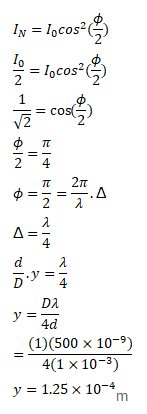# In a Young's double slit experimentQuestion:

In a Young's double slit experiment $\lambda=500 \mathrm{~nm}, \mathrm{~d}=1.0 \mathrm{~mm}$ and $D=1.0 \mathrm{~m}$. Find the minimum distance from the central maximum for which the intensity is half of the maximum intensity.

Solution: